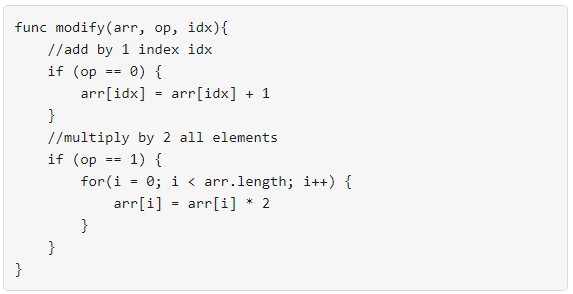# GeetCode HubYour task is to form an integer array `nums` from an initial array of zeros `arr` that is the same size as `nums`.

Return the minimum number of function calls to make `nums` from `arr`.

The answer is guaranteed to fit in a 32-bit signed integer.

Example 1:

```Input: nums = [1,5]
Output: 5
Explanation: Increment by 1 (second element): [0, 0] to get [0, 1] (1 operation).
Double all the elements: [0, 1] -> [0, 2] -> [0, 4] (2 operations).
Increment by 1 (both elements)  [0, 4] -> [1, 4] -> [1, 5] (2 operations).
Total of operations: 1 + 2 + 2 = 5.
```

Example 2:

```Input: nums = [2,2]
Output: 3
Explanation: Increment by 1 (both elements) [0, 0] -> [0, 1] -> [1, 1] (2 operations).
Double all the elements: [1, 1] -> [2, 2] (1 operation).
Total of operations: 2 + 1 = 3.
```

Example 3:

```Input: nums = [4,2,5]
Output: 6
Explanation: (initial)[0,0,0] -> [1,0,0] -> [1,0,1] -> [2,0,2] -> [2,1,2] -> [4,2,4] -> [4,2,5](nums).
```

Example 4:

```Input: nums = [3,2,2,4]
Output: 7
```

Example 5:

```Input: nums = [2,4,8,16]
Output: 8
```

Constraints:

• `1 <= nums.length <= 10^5`
• `0 <= nums[i] <= 10^9`

class Solution { public int minOperations(int[] nums) { } }